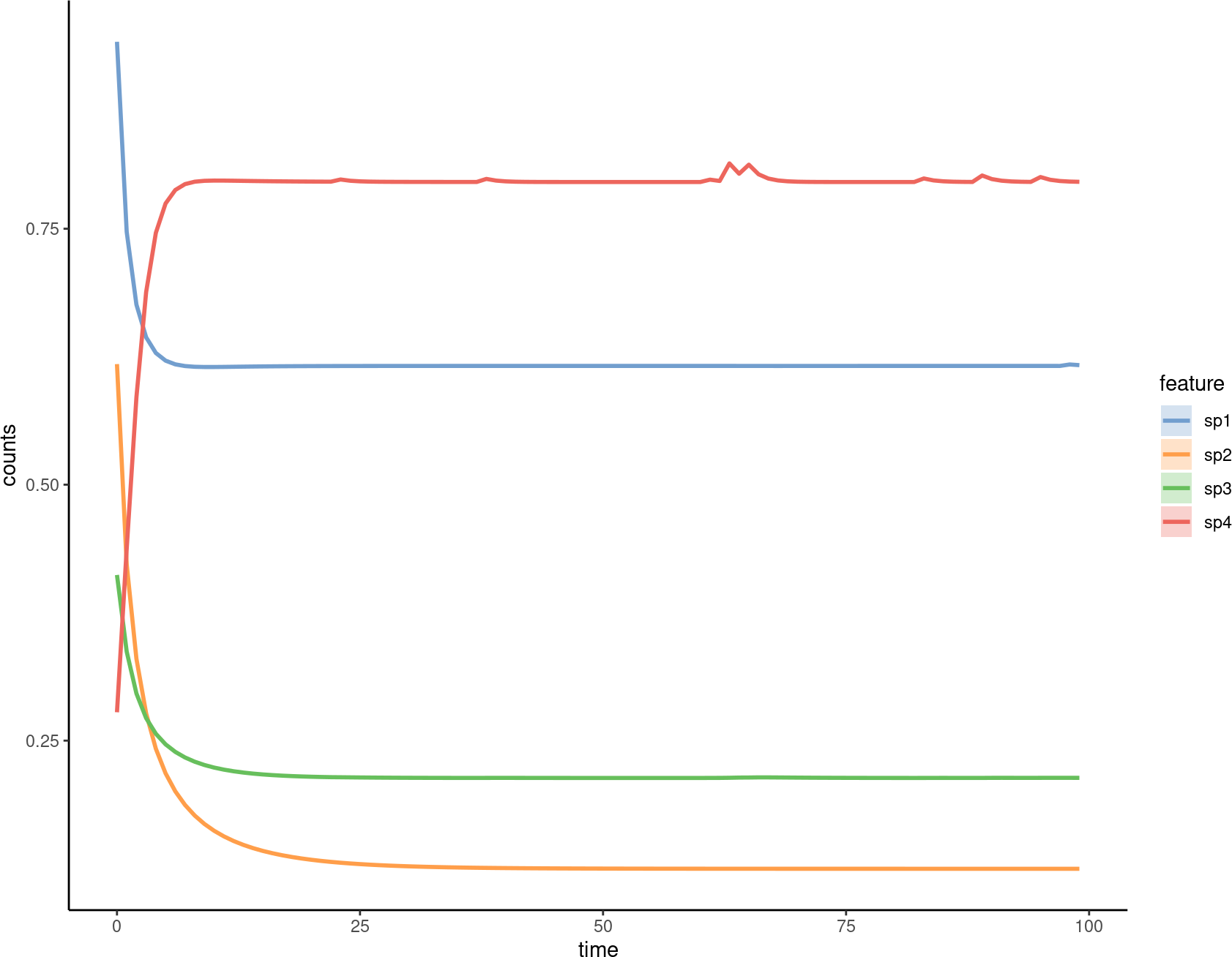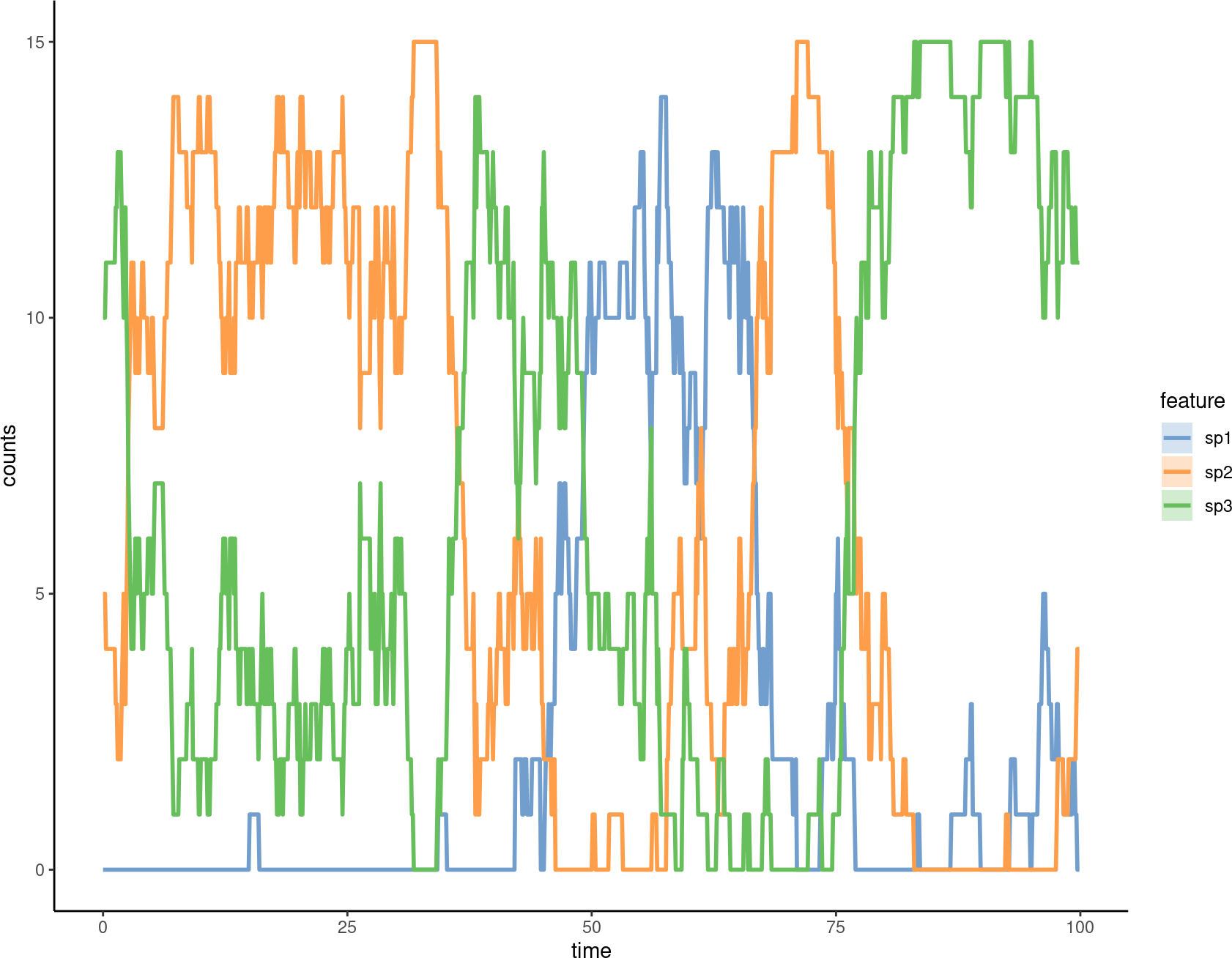# 1 Introduction

`miaSim` implements tools for simulating microbial community data based on various ecological models. These can be used to simulate species abundance matrices, including time series. A detailed function documentation can be viewed at the function reference page

## 1.1 Installation

Install the Bioconductor release version with

``````if (!requireNamespace("BiocManager", quietly = TRUE))
install.packages("BiocManager")``````

``library(miaSim)``

## 1.2 Examples

### 1.2.1 Generating species interaction matrices

Some of the models rely on interaction matrices that represents interaction heterogeneity between species. The interaction matrix can be generated with different distributional assumptions.

Generate interactions from normal distribution:

``A_normal <- powerlawA(n_species = 4, alpha = 3)``

Generate interactions from uniform distribution:

``A_uniform <- randomA(n_species = 10, diagonal = -0.4, connectance = 0.5, interactions = runif(n = 10^2, min = -0.8, max = 0.8))``

### 1.2.2 Generalized Lotka-Volterra (gLV)

The generalized Lotka-Volterra simulation model generates time-series assuming microbial population dynamics and interaction.

``````glvmodel <- simulateGLV(n_species = 4, A = A_normal, t_start = 0,
t_store = 100, t_end=100, stochastic = FALSE, norm = FALSE)

miaViz::plotSeries(glvmodel, "time")``````### 1.2.3 Ricker model

Ricker model is a discrete version of the gLV:

``rickermodel <- simulateRicker(n_species=4, A = A_normal, t_end=100, norm = FALSE)``

The number of species specified in the interaction matrix must be the same as the species used in the models.

### 1.2.4 Hubbell model

Hubbell Neutral simulation model characterizes diversity and relative abundance of species in ecological communities assuming migration, births and deaths but no interactions. Losses become replaced by migration or birth.

``````hubbellmodel <- simulateHubbell(n_species = 8, M = 10, carrying_capacity = 1000,
k_events = 50, migration_p = 0.02, t_end = 100)``````

One can also simulate parameters for the Hubbell model.

``````hubbellmodelRates <- simulateHubbellRates(x0 = c(0,5,10),
migration_p = 0.1, metacommunity_probability = NULL, k_events = 1,
growth_rates = NULL, norm = FALSE, t_end=100)

miaViz::plotSeries(hubbellmodelRates, "time")``````### 1.2.5 Self-Organised Instability (SOI)

The Self-Organised Instability (SOI) model generates time series for communities and accelerates stochastic simulation.

``````soimodel <- simulateSOI(n_species = 4, carrying_capacity = 1000,
A = A_normal, k_events=5, x0 = NULL, t_end = 150, norm = TRUE)``````

### 1.2.6 Stochastic logistic model

Stochastic logistic model is used to determine dead and alive counts in community.

``````logisticmodel <- simulateStochasticLogistic(n_species = 5)

miaViz::plotSeries(logisticmodel, x = "time")

model_transformed <- mia::transformCounts(logisticmodel, method = "relabundance")``````

### 1.2.7 Consumer-resource model

The consumer resource model requires the use of the `randomE` function, which returns a matrix containing the production rates and consumption rates of each species. The resulting matrix is used as a determination of resource consumption efficiency.

``````crmodel <- simulateConsumerResource(n_species = 2,
n_resources = 4,
E = randomE(n_species = 2, n_resources = 4))

miaViz::plotSeries(crmodel, "time")

# example to get relative abundance and relative proportion of resources
#'norm = TRUE' can be added as a parameter.

# convert to relative abundance
ExampleCR <- mia::transformCounts(crmodel, method = "relabundance")

miaViz::plotSeries(ExampleCR, "time")``````
``````#Recommended standard way to generate a set of n simulations (n=2 here) from a given model
simulations <- lapply(seq_len(2), function (i) {do.call(simulateConsumerResource, params)})

# Visualize the model for the first instance
miaViz::plotSeries(simulations[], "time")

# List state for each community (instance) at its last time point;
# this results in instances x species matrix; means and variances per species can be computed col-wise

communities <-  t(sapply(simulations, function (x) {assay(x, "counts")[, which.max(x\$time)]}))

# Some more advanced examples for hardcore users:

# test leave-one-out in CRM
.replaceByZero <- function(input_list) { # params_iter\$x0 as input_list
if (!all(length(input_list) == unlist(unique(lapply(input_list, length))))) {
stop("Length of input_list doesn't match length of element in it.")
}
for (i in seq_along(input_list)) {
input_list[[i]][[i]] <- 0
}
return(input_list)
}

.createParamList <- function(input_param, n_repeat, replace_by_zero = FALSE) {
res_list <- vector(mode = "list", length = n_repeat)
for (i in seq_len(n_repeat)) {
res_list[[i]] <- input_param
}
res_list <- lapply(seq_len(n_repeat), function (i) {input_param})
}``````
``````# example of generateSimulations
# FIXME: reduce computational load by lowering the number of species and timesteps in the demo
params <- list(
n_species = 10,
n_resources = 5,
E = randomE(
n_species = 10, n_resources = 5,
mean_consumption = 1, mean_production = 3
),
x0 = rep(0.001, 10),
resources = rep(1000, 5),
monod_constant = matrix(rbeta(10 * 5, 10, 10), nrow = 10, ncol = 5),
inflow_rate = .5,
outflow_rate = .5,
migration_p = 0,
stochastic = TRUE,
t_start = 0,
t_end = 20,
t_store = 100,
growth_rates = runif(10),
norm = FALSE
)
# Test overwrite params
.createParamList <- function(input_param, n_repeat, replace_by_zero = FALSE) {
res_list <- unname(as.list(data.frame(t(matrix(rep(input_param, n_repeat), nrow = n_repeat)))))
}

paramx0 <- .createParamList(input_param = rep(0.001, 10), n_repeat = 10,
replace_by_zero = TRUE)
paramresources <- .createParamList(input_param = rep(1000, 5), n_repeat = 10)
params_iter <- list(x0 = paramx0, resources = paramresources)
simulations <- lapply(seq_len(2), function (i) {do.call(simulateConsumerResource, params)})
simulations_2 <- .generateSimulations(
model = "simulateConsumerResource",
params_list = params, param_iter = params_iter, n_instances = 1, t_end = 20
)

estimatedA <- .estimateAFromSimulations(simulations, simulations_2, n_instances = 1,
scale_off_diagonal = 1, diagonal = -0.5, connectance = 0.2
) / 1000
# Using these parameters with a specified simulator
m <- simulateGLV(n_species = 10, x0 = params\$x0,
A = estimatedA, growth_rates = params\$growth_rates, t_end = 20, t_store = 100)

miaViz::plotSeries(m, "time") # Plotting``````